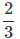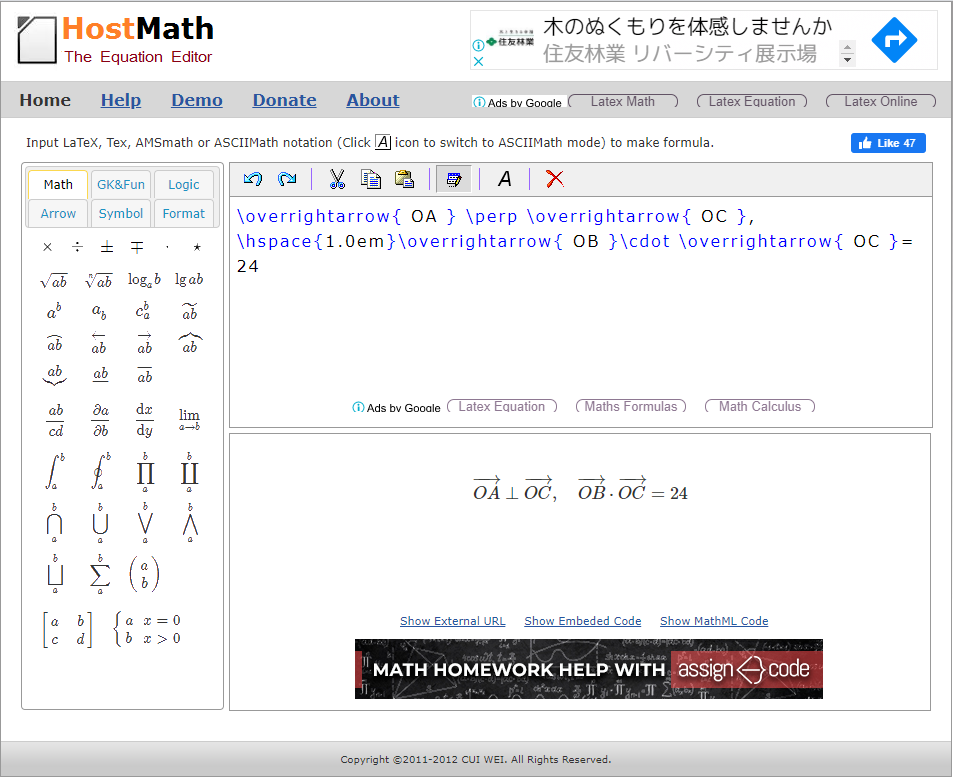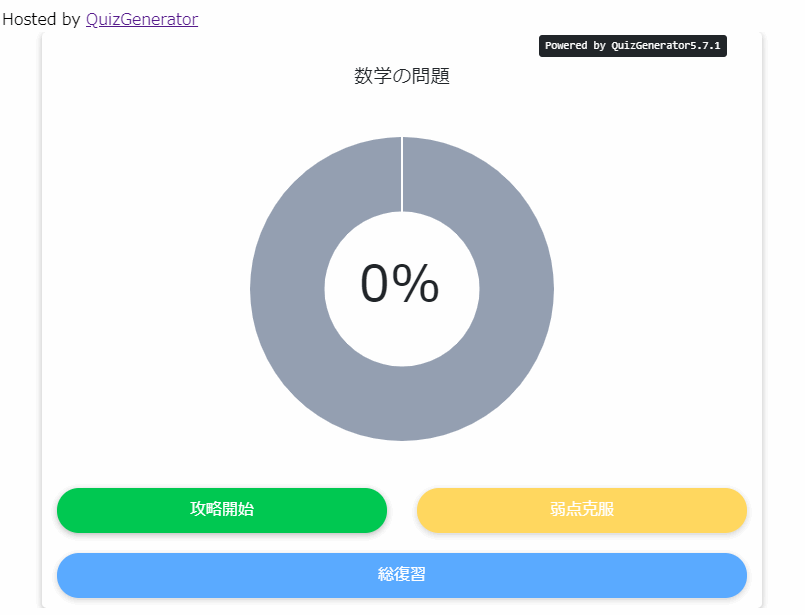QuizGenerator

# QuizGenerator is an e-learning system that supports mathematical formulas and special symbols.

Hello, I'm Kobashi from development🌵Our e-learning system is flexible to mathematical formulas, which are used in math problems. In this article, I will explain how to use mathematical formulas, equations, and special symbols.How to use "TeX" to create a beautiful notation without losing its appearance.We are pleased to introduce the

Ladies and gentlemen.Have you ever had a problem creating a math problem on the web?When creating a math problem, the most annoying part isEntering formulasI know, right? I have experienced a great deal of trouble finding how to input roots and fractions when I input mathematical expressions (>_<) There are many complicated formulas such as logarithms (log) and sigma (sigma) as well as fractions and roots. Please refer to this paper and try to notate beautiful formulas.

Contents

• 1. what is TeX?
• 3. I made a math problem for the 2020 Center Test.
• 4. summary

## What is "TeX" anyway?It is an electronic typesetting system developed by Donald E. Knuth. The pronunciation of "TeX" is difficult for Japanese people."Teff.It seems to be called
Numbers and letters can be arranged beautifully with detailed layout adjustments such as spacing, line spacing, and size. It is also excellent as a function for displaying mathematical expressions, and even seemingly difficult mathematical expressions and special mathematical characters can be output beautifully with smooth lines. You can use it for a variety of notations, not just mathematical expressions, but we will focus on "mathematical expressions" here.

It is recommended to type TeX notation as it is, because it is quicker.

HostMathHostMathallows you to preview the converted formula in real time every time you change a part of the prepared TeX notation. You can easily paste and combine multiple formulas and it is very easy to use. Unfortunately, the number of available formulas is limited, so you may not find the formula you need.

### Our Tex confirmation tool is here

OurTex Verification Tool for QuizGeneratorWith nearly 400 formulas and symbols to choose from, you're sure to find the formula or special symbol you're looking for.

### Here's how to set it up if you use our Tex confirmation tool

As a promise, all TeX notations are \ (backslash)Start withSuperscript is ^, subscript is _ (underscore)in front of the number.

Example: If you want to write a fraction
fractionwhat? \frac{numerator}{denominator} The following notation is used ⇒.is\frac{2}{3}The result is the following.

Notes
Please note that when you use the learningBOX and QuizGenerator forms to create a question (as I am about to show you in the example), make sure that you use TeX notation before and after the[# #]Don't forget to enclose it with If you forget, the TeX notation will not be converted and will be displayed as it is (>_<).

## I created a math problem for the 2020 Center Test.

QuizGenerator/learningBOX supports TeX.
As an example, I took an excerpt from a mathematics problem of this year's center entrance examination and made it in learningBOX.

[Math II, Math B, Question 4]At first glance, the formula input seemed difficult, but I was able to create it rather smoothly! If you get used to it, you'll be able to create it quickly. The input contents are as follows. The blue part is TeX notation.

There are two points A(3, 3, -6) and B(2+2) in the coordinate space whose origin is the point O.[#\sqrt{3}#], 2-2[#\sqrt{3}#], -4) is taken.
Let a be the plane defined by the three points O, A and B. Also, the point C contained in a is

[#\overrightarrow{ OA }\perp \overrightarrow{ OC }, \hspace{1.0em}\overrightarrow{ OB }\cdot \overrightarrow{ OC }= 24#]
・・・・・・・・・・・・・・・・・・①

Suppose that we satisfy

(1) [#| \overrightarrow{ OA } |= \fbox{a}\sqrt{\fbox{i}}#] and
[#| \overrightarrow{ OB } |= \fbox{u}\sqrt{\fbox{e}}#] and
[#\overrightarrow{ OA }\cdot \overrightarrow{ OB } = \fbox{Oca}#] is.

Of course, I didn't study TeX notation and type it in; I just copied the one generated by HostMath with [##] around it and pasted it into the question form.This kind of formula is also easy to input.By the way, you can also specify text style such as coloration and text size in TeX notation.

\tiny,\small,\normal,\large,\huge
For example, just add it just before the application point as shown below. If you don't specify the size, it will be the same size as \normal.
You can also use {} to enclose the area where you want to adjust the color.
Below is an example of adjusting the size and color.\blur1}small\color{red} {\frac{-b\pm\sqrt{b^2-4ac}}{2a}}
\large\color{green} {\frac{-b\pm\sqrt{b^2-4ac}}{2a}}
\fnDroid Sans Fallback\color{blue} {\frac{-b\pm\sqrt{b^2-4ac}}{2a}}

With ▼QuizGenerator, you can create quizzes with beautiful looking formulas!The math quiz is list of optionsIt can be solved from

## Summary

In this article, I briefly introduced TeX. Recently, there are some tools that can convert handwriting and images. However, if you don't have a pen for input, it's sluggish to write while pulling your hand with a mouse. It's also troublesome to prepare images one by one. If you don't have a pen, you can use a mouse. In such a case, it is recommended to type the TeX notation as it is.
From now on, I can use TeX to create problems with QuizGenerator/learningBOX without being afraid of any formulas, right?
Trigonometric functions such as \sin and logarithmic functions such as \log are also provided, so you can write basic TeX notation intuitively without referring to anything in particular, and you don't have to learn TeX notation for complex notation by using the automatic generation tool. Please get acquainted with TeX and try to write beautiful mathematical expressions.